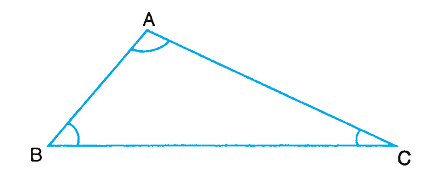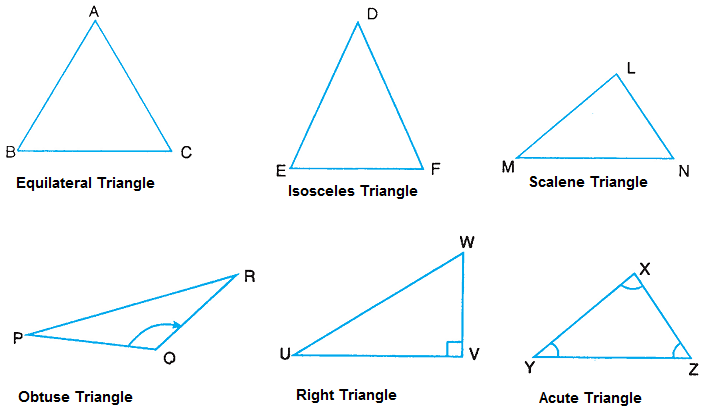# Types of Triangle

Triangle is the simplest polygon of all the closed figures formed in a plane by three line segments. It is a closed figure formed by three line segments having six elements - three angles (i) ∠ABC or ∠B (ii) ∠ACB or ∠C (iii) ∠CAB or ∠A and three sides - (iv) AB (v) BC (vi) CA.It is named as Δ ABC or Δ BAC or Δ CBA and read as triangle ABC or triangle BAC or triangle CBA.

### Types of Triangles

Triangles can be classified into different types in two ways:

On the basis of sides

1. Equilateral triangle: A triangle in which all the three sides are equal
2. Isosceles triangle: A triangle in which two sides are equal
3. Scalene triangle: A triangle in which all sides are of different lengths

On the basis of angles

1. Obtuse angled triangle: A triangle in which one of the angles is an obtuse angle
2. Right angled triangle: A triangle in which one of the angles is a right angle
3. Acute angled triangle: A triangle in which all the three angles are acute